# PERCENTILE Excel FunctionArticle byExcelMojo Team## What Is PERCENTILE Excel Function?

The PERCENTILE Excel function returns the kth percentile of the given range of values based on its position in a dataset. For improved accuracy, PERCENTILE() in Excel 2010 was replaced with PERCENTILE.INC(). Therefore, the PERCENTILE() is now available in Excel as a Compatibility function for backward compatibility.

The PERCENTILE Excel function is an inbuilt function so that we can insert the formula from the “Function Library” or enter it directly in the worksheet. For example, the below image shows two tables. The first table contains a candidate list and their height details. And in the second table, we can calculate the various percentiles below which the specified percentages of candidates in table one fall, based on their heights.

Select cell G3, enter the formula =PERCENTILE(\$B\$2:\$B\$11,E3), and press the “Enter” key.

Drag the formula from cell G3 to G7 using the fill handle.

The output is shown above.

[Output Observation: In cell G3, the function inputs are the height values range B2:B11 and the required percentile value, 0.2. And it returns the percentile value as 165.8 cm, which implies that 20% of the candidates have heights below 165.8 cm.]

###### Key Takeaways
• The PERCENTILE Excel function is a Statistical function that determines the kth percentile of the supplied range of data.
• The function PERCENTILE.INC replaced the PERCENTILE() in Excel 2010. However, the PERCENTILE() is still available in Excel as a Compatibility function for backward compatibility.
• We must enter the kth percentile as a decimal value, i.e., 30% as 0.3, 75% as 0.75, and so on.
• We can use the PERCENTILE() with IF() when determining the kth percentile involving specific conditions. When we use the IF() function, we use the keys Ctrl + Shift + Enter to execute as an array formula.

### PERCENTILE() Excel Formula

The syntax of the PERCENTILE Excel formula is:

The arguments of the PERCENTILE Excel formula are:

• array: The cell range or array of values or data points defining the relative standing. It is a mandatory argument.
• k: The percentile value between 0 and 1, inclusive. It is a mandatory argument.

### How to Use PERCENTILE Excel Function?

We can use the PERCENTILE Excel function in 2 methods, namely,

1. Access from the Excel ribbon.
2. Enter in the worksheet manually.

#### Method #1 – Access from the Excel ribbon

First, choose an empty cell → select the “Formulas” tab → go to the “Function Library” group → click the “More Functions” options drop-down → click the “Compatibility” option right arrow à select the “PERCENTILE” function, as shown below.

The “Function Arguments” window appears. Enter the argument values in the “Array” and “K” fields → click “OK”, as shown below.

#### Method #2 – Enter in the worksheet manually

1. Select an empty cell for the output.
2. Type =PERCENTILE( in the cell. [Alternatively, type =P and select the PERCENTILE function from the Excel suggestions.]
3. Enter the arguments as values or cell references in Excel and close the brackets.
4. Press the “Enter” key.

Let us take an example to understand this funciton.

We will find the 25th PERCENTILE of the student scores using PERCENTILE Excel function.

The following table shows a list of students and their scores in Mathematics.

The procedure to find the 25th PERCENTILE using PERCENTILE Excel function is:

1. Select cell B14, enter the formula =PERCENTILE(B2:B11,0.25), and press the “Enter” key.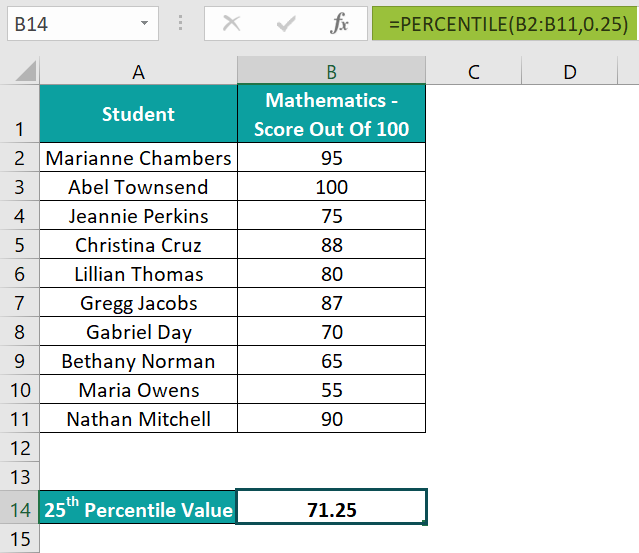The output is shown above.

[Output Observation: Here, the first argument is the array range of scores, B2:B11, and the second argument, k, is in the decimal format, 0.25. And the 25th PERCENTILE Excel function output is 71.25. So, it implies that 25% of the students have scored less than 71.25.]

2. [Alternatively: Select the target cell B14 and click Formulas → More Functions → Compatibility → PERCENTILE to open the Function Arguments window.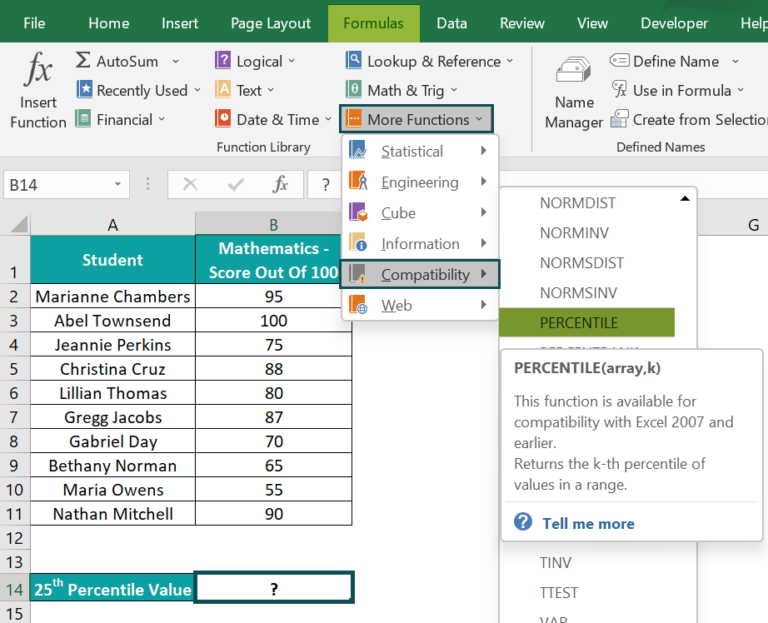3. Next, fill the Array and K fields in the Function Arguments window.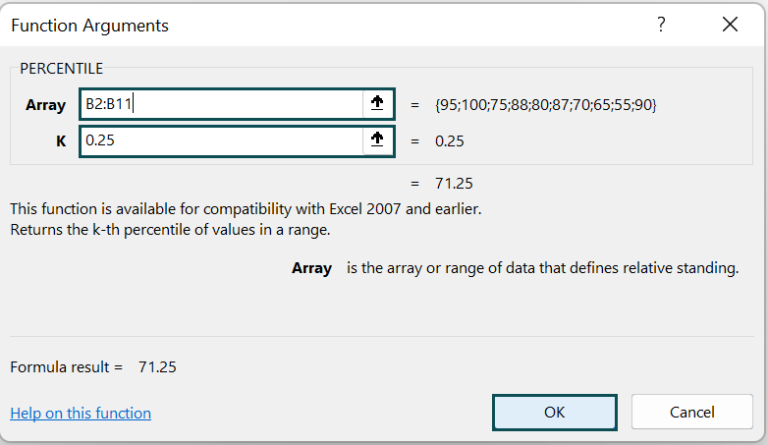And once we click OK in the Function Arguments window, the PERCENTILE() in the target cell B14 will get executed.]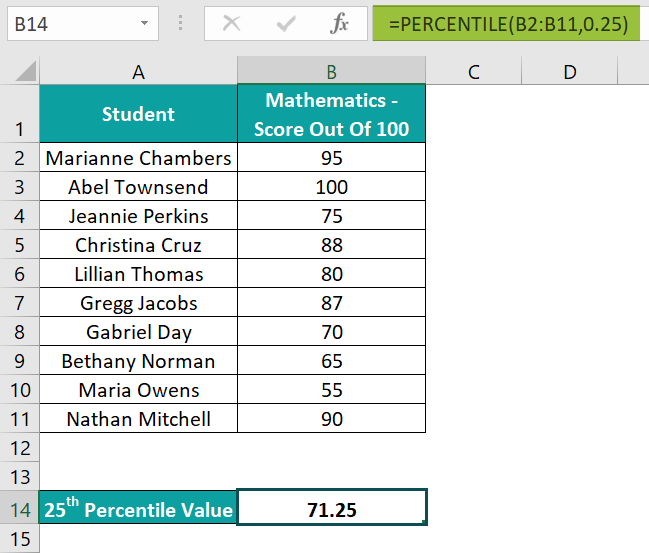### Examples

We will understand some advanced scenarios with PERCENTILE Excel function examples.

#### Example #1

This example shows how the PERCENTILE Excel function behaves when the second argument, k, is and is not a multiple of 1/(n-1), where n is the input array size. We will find the 40thand 50th percentiles for the list of applicants.

The following table contains a list of applicants and their ages.

The steps to find the 40th and 50th percentile using the PERCENTILE Excel function are:

• Step 1: Select cell F1, enter the formula =PERCENTILE(B2:B7,0.4), and press the “Enter” key.

[Here, the second argument is 0.4 (40% is decimal form), and the value of 1/(n-1) is 0.2, as n is 6 (the size of the input array B2:B7). As 0.4 is a multiple of 0.2, the PERCENTILE() does not have to interpolate to find the value and the 40th percentile, and it returns the required percentile, 53, as is.]

• Step 2: Select cell F2, enter the formula =PERCENTILE(B2:B7,0.5), and press the “Enter” key.

[In this case, the 1/(n-1) value remains the same, 0.2. However, the k value, 0.5, is not a multiple of 0.2. So, the 50th percentile falls midway between the values 53 and 54, and the PERCENTILE() insinuates to determine the output 53.5.]

#### Example #2

We will find the 50th percentile of the range of average household income values for the state CA PERCENTILE function with the IF Excel function.

The below table contains the list of the top 10 richest cities in the US, based on the average household income.

The steps to use the PERCENTILE Excel function and the IF() functions are:

• Step 1: Select cell G2, enter the formula =PERCENTILE(IF(C2:C11=F2,B2:B11),0.5), and execute it as an array formula in Excel using the keys Ctrl + Shift + Enter. The output is shown below.

[Note: The formula then will become {=PERCENTILE(IF(C2:C11=F2,B2:B11),0.5)}, i.e., an array formula. Since the IF() contains an array range, we must apply the formula as an array formula.]

• Step 2: Select cell G2 and set the Number Format option in the Home tab as Currency to view the result as a currency value.

The output in the array format is shown below.

[Output Observation: First, the IF() condition is {CA;NY;CO;CA;CA;NJ;TX;CT;NY;IL}=CA, which translates as {TRUE;FALSE;FALSE;TRUE;TRUE;FALSE;FALSE;FALSE;FALSE;FALSE}. Then, the TRUE values get updated with the corresponding currency values in the array range, {450696;FALSE;FALSE;386174;373128;FALSE;FALSE;FALSE;FALSE;FALSE}.

Finally, the PERCENTILE() determines the 50th percentile of the average household income values in the array range {450696,386174,373128} for CA to return the value 386174.]

#### Example #3

We shall now see PERCENTILE.INC(), the replacement for the PERCENTILE Excel function.

The PERCENTILE.INC() syntax is:

The arguments’ definitions and the function logic are the same as PERCENTILE(), but with improved accuracy.

We will determine the monthly salary below which the monthly salaries of 90% of the employees fall, and compare the two functions. The below table contains a list of employees and their monthly salary details.

The steps to apply PERCENTILE.INC() are as follows:

• Step 1: Select cell B15, enter the formula =PERCENTILE.INC(B2:B11,90%), and press the “Enter” key.
• Step 2: Select cell B16, enter the formula =PERCENTILE(B2:B11,90%), and press the “Enter” key.
• Step 3: Select cell B15:B16 and set the Number Format in the Home tab as Currency.

The output is shown below. Thus, both, PERCENTILE.INC() and PERCENTILE Excel function show that 90% of the employees have monthly salaries below \$6,320.00.

### Important Things to Note

• If the second argument, k, is non-numeric, the PERCENTILE Excel function gives a #VALUE! error.
• For a k<0, k>1, or an empty array, the PERCENTILE() returns the #NUM! error.
• For an input array size n, if k is not a multiple of 1/(n-1), the PERCENTILE() insinuates to determine the kth percentile value.

1. Does Excel have a PERCENTILE function?

Excel has a PERCENTILE function in the Formulas tab. Click Formulas More Functions CompatibilityPERCENTILE to insert the function in the required target cell.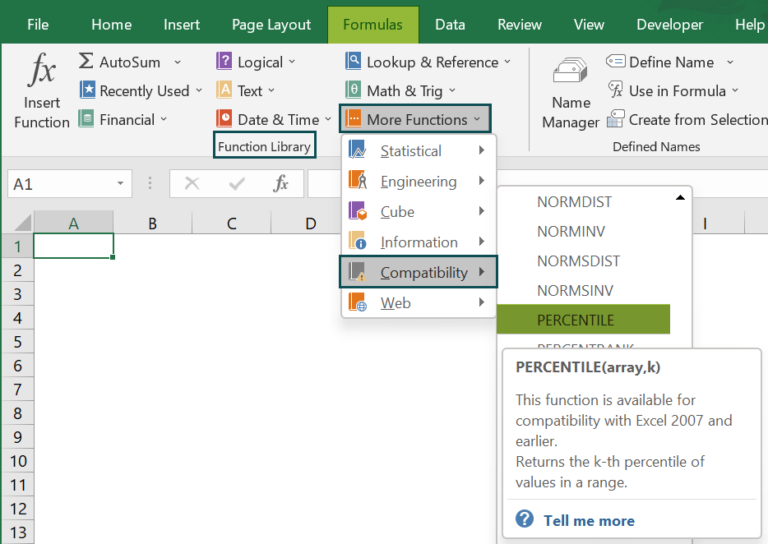2. How do I calculate the 95% percentile in Excel?

We can calculate the 95% percentile in Excel using the PERCENTILE().

For example, the below table shows the data for units of a commodity delivered each month.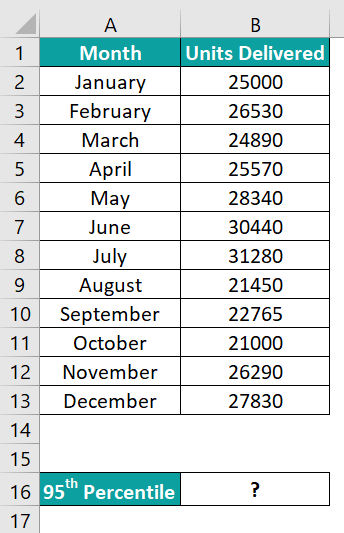Select cell B16, enter the formula =PERCENTILE(B2:B13,95%), and press the “Enter” key.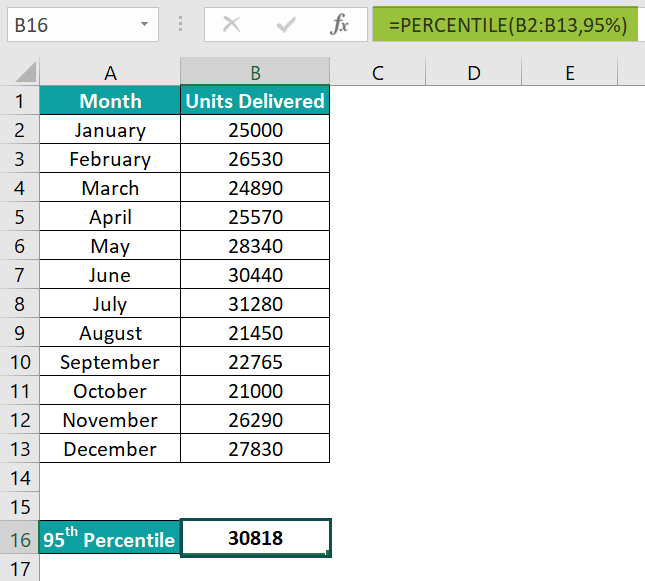The output is shown above.

[Alternatively, we can enter the formula =PERCENTILE(B2:B13,0.95) to get the same results.]

3. How to use the PERCENTILE Excel function with multiple conditions?

We can use the PERCENTILE Excel function with multiple conditions using the IF() function.

For example, the below image contains a list of students and their details. We must find the aggregate above which the top 10% of the students have their aggregates, who are on the prefectorial board and are also rank holders, i.e., the 90th percentile.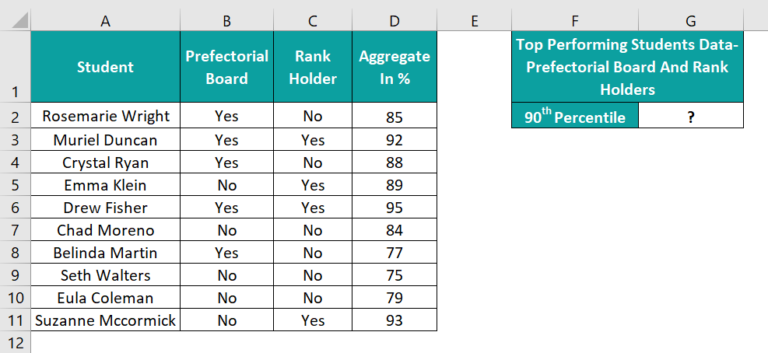Select cell G2, enter the formula =PERCENTILE(IF((B2:B11=”Yes”)*(C2:C11=”Yes”),D2:D11),0.9)} and press Ctrl + Shift + Enter keys to execute the function as an array formula.

The formula will now be {=PERCENTILE(IF((B2:B11=”Yes”)*(C2:C11=”Yes”),D2:D11),0.9)}The output is shown above.

[Output Observation: First, the IF() checks the arrays B2:B11 and C2:C11 for Yes values to determine students on the prefectorial board and who are rank holders. And so, the IF condition array becomes {0;1;0;0;1;0;0;0;0;0}, indicating that two students, Muriel Duncan and Drew Fisher, satisfy both the conditions. Thus, the IF() returns the corresponding column D values, 92 and 95.

Finally, the PERCENTILE() accepts the array argument as {92,95} and determines the 90th percentile to return the value 94.7. The result shows that the top 10% of the students satisfying the above conditions have aggregates above 94.7%.]